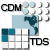## Dataset: Aggregated 7 day CIOFS Fields Forecast/Best Time Series

• Data format: netCDF
• Data type: GRID
• Naming Authority: gov.noaa.nos.co-ops
• ID: CIOFS/fmrc/Aggregated_7_day_CIOFS_Fields_Forecast_best.ncd

### Variables:

• Vocabulary [CF-1.0]:
• Pair (millibar) = surface air pressure = surface_air_pressure
• Uwind (meter second-1) = surface u-wind component = grid_eastward_wind
• Vwind (meter second-1) = surface v-wind component = grid_northward_wind
• angle (radians) = angle between XI-axis and EAST =
• f (second-1) = Coriolis parameter at RHO-points =
• h (meter) = bathymetry at RHO-points = sea_floor_depth
• pm (meter-1) = curvilinear coordinate metric in XI =
• pn (meter-1) = curvilinear coordinate metric in ETA =
• salt = salinity = sea_water_salinity
• temp (Celsius) = potential temperature = sea_water_temperature
• u (meter second-1) = u-momentum component = grid_eastward_sea_water_velocity
• v (meter second-1) = v-momentum component = grid_northward_sea_water_velocity
• w (meter second-1) = vertical momentum component = vertical_sea_water_velocity
• zeta (meter) = free-surface = sea_surface_height

### GeospatialCoverage:

• Longitude: -156.48529100000002 to -148.92512499999998 degrees_east
• Latitude: 56.7004919 to 61.5247774 degrees_north
• Altitude: -1.0 to 0.0 Resolution=0.03333333333333333 (positive is down)

### TimeCoverage:

• Start: 2020-07-29T19:00:00Z
• End: 2020-08-08T18:00:00Z